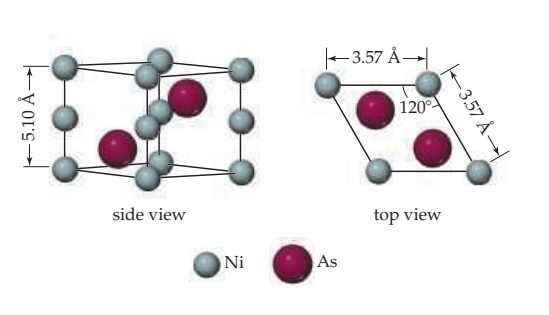×
Get Full Access to Chemistry: The Central Science - 14 Edition - Chapter 12 - Problem 12.29
Get Full Access to Chemistry: The Central Science - 14 Edition - Chapter 12 - Problem 12.29

×

# ?The unit cell of nickel arsenide is shown here. (a) What type of lattice does this crystal possess? (b) What is the empirical formISBN: 9780134414232 1274

## Solution for problem 12.29 Chapter 12

Chemistry: The Central Science | 14th Edition

• Textbook Solutions
• 2901 Step-by-step solutions solved by professors and subject experts
• Get 24/7 help from StudySoup virtual teaching assistantsChemistry: The Central Science | 14th Edition

4 5 1 318 Reviews
16
4
Problem 12.29

The unit cell of nickel arsenide is shown here.

(a) What type of lattice does this crystal possess?

(b) What is the empirical formula?Step-by-Step Solution:

Step 1 of 5) A strong acid completely transfers its protons to water, leaving essentially no undissociated molecules in solution. (Section 4.3) Its conjugate base has a negligible tendency to accept protons in aqueous solution. (The conjugate base of a strong acid shows negligible basicity.) 2. A weak acid only partially dissociates in aqueous solution and therefore exists in the solution as a mixture of the undissociated acid and its conjugate base. The conjugate base of a weak acid shows a slight ability to remove protons from water. (The conjugate base of a weak acid is a weak base.) 3. A substance with negligible acidity contains hydrogen but does not demonstrate any acidic behavior in water. Its conjugate base is a strong base, reacting completely with water, to form OH- ions. (The conjugate base of a substance with negligible acidity is a strong base.)The ions H3O+1aq2 and OH- 1aq2 are, respectively, the strongest possible acid and strongest possible base that can exist at equilibrium in aqueous solution. Stronger acids react with water to produce H3O+1aq2 ions, and stronger bases react with water to produce OH- 1aq2 ions, a phenomenon known as the leveling effect.

Step 2 of 2

##### ISBN: 9780134414232

This full solution covers the following key subjects: . This expansive textbook survival guide covers 29 chapters, and 2820 solutions. This textbook survival guide was created for the textbook: Chemistry: The Central Science, edition: 14. Since the solution to 12.29 from 12 chapter was answered, more than 217 students have viewed the full step-by-step answer. Chemistry: The Central Science was written by and is associated to the ISBN: 9780134414232. The answer to “?The unit cell of nickel arsenide is shown here. (a) What type of lattice does this crystal possess? (b) What is the empirical formula?” is broken down into a number of easy to follow steps, and 24 words. The full step-by-step solution to problem: 12.29 from chapter: 12 was answered by , our top Chemistry solution expert on 10/03/18, 06:29PM.

## Discover and learn what students are asking

Calculus: Early Transcendental Functions : Riemann Sums and Definite Integrals
?In Exercises 3–8, evaluate the definite integral by the limit definition. $$\int_{-1}^{1} x^{3} d x$$

Calculus: Early Transcendental Functions : Inverse Trigonometric Functions: Integration
?In Exercises 1-20, find the indefinite integral. $$\int \frac{1}{x \sqrt{x^{4}-4}} d x$$

Statistics: Informed Decisions Using Data : Measures of Position and Outliers
?Explain what each quartile represents.

Unlock Textbook Solution